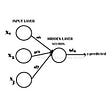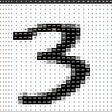# Neural Networks From Scratch (NNFS) Chapter 9: Backpropagation

I am writing this as supplementary material for the book Neural Networks From Scratch, as I’ve noticed that many people have a hard time (as I did) with chapter 9 which covers backpropagation. I just want to offer some (hopefully) helpful visualizations that helped me understand what was happening here. This is not a finished draft, as I haven’t even finished the book yet, so feel free to suggest edits and/or point out errors I’ve made.

As a disclaimer, I am NOT the author of Neural Networks from Scratch. I am but a humble reader <3

Starting on page 204

So far we have reduced the output of a single neuron, which has received inputs from 3 neurons in the previous layer, which looked like this.

Now, in order to perform a successful backpropagation, we (basically) need to reverse engineer our forward pass. In order to really get a grasp on how to do that, let’s consider this visualization from a different perspective.

Let’s imagine that we have a dataset containing 50 samples and 3 features-per-sample. What would this matrix look like?

And we’re going to pass this dataset through a single dense layer containing 2 neurons. What would the “weights” matrix look like in this single dense layer?

When we perform our forward pass, we perform matrix multiplication, let’s think about exactly what happens during that process. Let’s first make sure that our dimensions match.

We can see that the correct dimensions match. So what will be the result?

The resulting matrix shape will be the first dimension of the first matrix, and the second dimension of the second matrix. The resulting matrix will then become the output of the following layer.

Let’s now add these into our visualizations of the network to combine our conceptual understanding of a network, with our mathematical understanding of what’s happening in our code.

For an added layer of understanding, let’s add the shapes of these matrices into our visualization to see what must match. Remember, the green must match and the purple is the result.

The result of this matrix multiplication will become the outputs for Layer 2.

We will ignore the bias for right now in order to keep this train-of-though as simple as possible.

Let’s now add our ReLU activation function onto the outputs at layer 2. This function obviously will not change the shape of our layer 2 outputs at all, as it is simply a max function.

We have now performed all of our operations which have lead to the outputs of layer 2. Let’s add some consistency to our diagram by replacing our multiplication with a function.

Now, let’s practice backpropagation by attempting to reduce the output of layer 2. To start, we can look at the expression that gave us our output and find the derivative from there.

Now find the derivative of this expression with respect to weights. Well this is actually pretty easy since we’ve simplified our expression. It’s going to look like this:

Let’s use the rules we already know about max function derivatives, and product derivatives, to simplify this expression with respect to weights.

At this point, we might be able to understand what we’re doing mathematically, but let’s go back to a matrix diagram to see what’s literally about to happen.

As you can see, we have a problem. We need to multiply these matricies together, but our dimensions do not match! At this point, we’re basically doing what we did in the forward pass, just backwards.

Now let’s think… we’re solving with respect to weights… and whatever the partial derivative is, it’s going to have the same shape as the weights… let’s look at the shape of the weights again to see what our partial derivative (aka the result of our operation) is supposed to look like.

Ok… now let’s see what this has in common with our equation so far…

Ahh ha! Well would you look at that… our equation so far has all the makings of our desired output, things just seem to be out of order. Let’s transpose our Inputs and swap the order of the matrix multiplication (i.e. Inputs * ReLU(Output) instead of ReLU(Output) * Inputs). The following is a visual representation of the transformation we’re going to make.

And after the transformation.

Great! We now having matching dimensions. Let’s look at what the result of this matrix multiplication will be now.

Awesome! Now when we perform this matrix multiplication the result will match our desired shape! Let’s take a look at an example of this in code.

In this example I take the mean of the output to confirm that the resulting matrices do, in fact, get reduced by our operation.

--

--

--

## More from Alec Mather

Developer 💻 || Writer 📝

Love podcasts or audiobooks? Learn on the go with our new app.

## Creating AI-powered computer vision applications in no time with IBM PowerAI Vision.## The Seinfeld## Cost Functions: The Underpinnings of Machine Learning## Deep Neural Networks With Python## Machine Learning Ball Case Study## How To Create Artistic Masterpieces With Deep Learning## Alec Mather

Developer 💻 || Writer 📝

## Training of Neural Networks — Back Propagation## Creating a Baseline Model for MNIST Dataset## 3 ways to create a Deep Neural Network model with Keras and Tensorflow 2.0## Fundamentals of Neural Networks